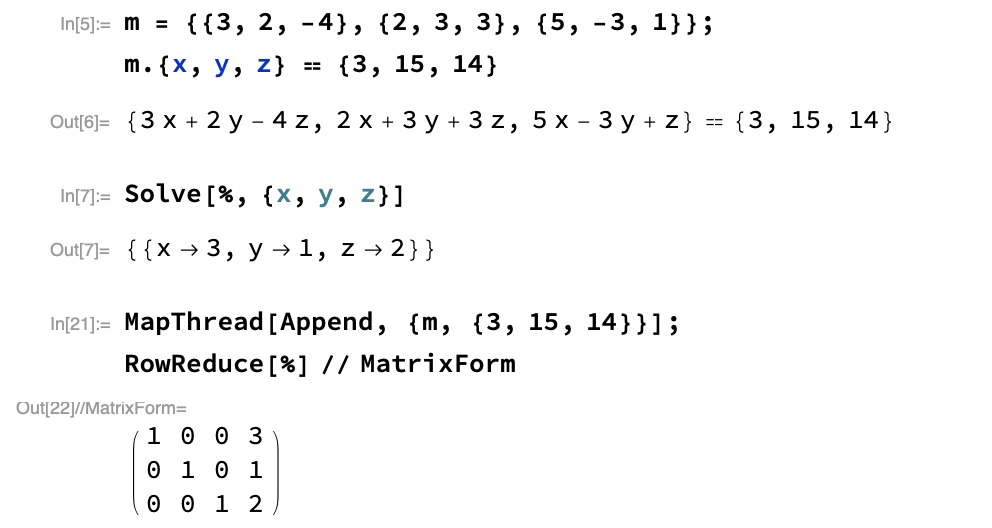# aliquot

## < a quantity that can be divided into another a whole number of time />

Gaussian elimination is probably one of the algorithm we hear about when taking a basic class in linear algebra. In essence, Gaussian elimination is a procedure that can solve $n$ linear equations in $n$ unknowns using on the order of $n^3$ arithmetic operations.1

How does it works? The idea is to transform a linear system, $Ax=b$, where $A$ is an $n\times n$ matrix and $x$ and $b$ are column vectors of size $n$, into an upper triangular system by applying simple linear transformation on the left, much like Householder triangularization in the case of QR factorization. Regarding operations that are permitted these are: swapping rows, adding one row onto another, and multiply every factor of one row with a constant. Consider the following example:

\begin{align} 3x + 2y - 4z & = \phantom{1}3\cr 2x + 3y + 3z & = 15\cr 5x - 3y + \phantom{3}z & = 14 \end{align}

You could do this by hand, by following the helpful tips from the site where this example originates from, but Mathematica has built-in procedures to solve linear systems like this, e.g. Solve or LinearSolve, and there’s also a RowReduce function which acts like Gaussian elimination. Here’s what we get:This is not the only algorithm used in matrix factorizations, of course. Indeed, modern statistical computing rely on LU decomposition or QR factorization. The latter is what used in R for linear regression models, for instance. The QR decomposition of a real square matrix $A$ is a decomposition of $A$ as $A=QR$, where $Q$ is an orthogonal matrix (i.e., $Q^TQ=I$) and $R$ in an upper triangular matrix. Furthermore, if $A$ is nonsingular, this decomposition is unique. Such a factorization can be found using the Gram-Schmidt procedure.

Solving linear systems is quite easy in Racket thanks to the math module. Here is one way to find the solution of the above system (which is mostly what we did in another post):

#lang racket
(require math/matrix)

(define A
(matrix [[3.0  2.0  -4.0]
[2.0  3.0  3.0]
[5.0  -3.0  1.0]]))
(define b (col-matrix [3.0 15.0 14.0]))
(matrix-solve A b)


Luckily, Racket also provides some row-based algorithms, including Gauss elimination. So here is what we get using matrix-gauss-elim:

(define Ab (matrix-augment (list A b)))
(matrix-gauss-elim Ab)


1. Lloyd N. Trefethen. Numerical Analysis. See also Pr. L. N. Trefethen’s website, which is full of free resources. ↩︎